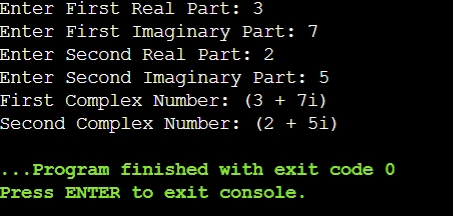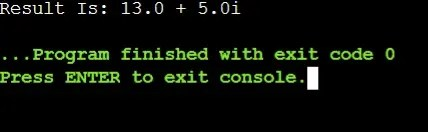# Java Program to Add Complex NumbersAre you searching for an article that will enlighten the “Java Program to Add Complex Numbers”? Are you not getting a concise article that will inform all the basic concepts of Complex numbers and a simple yet effective example to add complex numbers in a Java program?

Then, you have landed on the right page! In this article, we will first simply discuss the Mathematical Theory of Complex Numbers. Later, we will discuss the computer science approach to deal with any Complex Numbers using the Java programming language.

So, let us start one of the most intensive articles that will make a bridge between Computer Science and Mathematics.

We can understand that programming with mathematics can be a little difficult to understand, so you can always hire CodingZap experts if you need any assistance with your Java homework.

## What Is A Complex Number? Read Below

In Mathematics, there is a number system that divides every number into two parts. One is the Real Number that we all know like 1, 2, 3, etc. Another is the Complex Number which does not exist in nature but is present in only mathematical theory.The complex number can be again divided into two parts. The two parts of the complex numbers are known as Real and Imaginary parts. The real part of the complex number is made with the simple integer numbers. Whereas the imaginary part is denoted with a special symbol ‘i’.

General Symbol: a + bi [Real Part: ‘a’, Imaginary Part: ‘b’, Imaginary Symbol: ‘i’]

Example: 5 + 2i, 2 + 5i, 3 + 7i, etc.

## How To Add Real And Imaginary Parts Of The Complex Numbers Using Mathematical Concepts?

Here, we will decode the mathematical theory to add any two complex numbers. The addition of the complex numbers is done with the addition of Real and imaginary parts of two complex numbers. That means both Real Parts and Imaginary parts get added to each other.

If you wish to know more about mathematics and coding-related topics, you can check out our article on “Fibonacci Series”.

We can take one simple example to clarify the mathematical theory related to the addition of complex numbers with respect to Real and imaginary parts. Have a look at the following example:

```				```
Complex Number Equation 1: 5 + 2i........(1)
Complex Number Equation 2: 2 + 5i........(2)
In the above equations, the Real Parts are the 5 and 2 respectively.
In the above equations, the imaginary parts are the 2i and 5i respectively.
Now, addition of Real Parts: (5 + 2) = 7
Now, addition of Imaginary Parts: (2i + 5i) = 7i
The new complex number after addition of real and imaginary parts is: (7 + 7i)

```
```

So, one thing should be clear to you the addition of complex numbers means the addition of the real or imaginary part. It will be needed when we perform the addition of the real or imaginary part in our sample Java program.

But before that core concept, we need to know how to deal with complex numbers in any high-level programming language like Java.

## How To Create Complex Numbers in Java?

Now, we will first know about the creation of any complex number. Later, we will add the method that will help to add two complex numbers in the Java program. It is not the answer to the core topic, but it is the lead for that core discussion part.

Code to demonstrate the creation method of complex numbers in a Java program:

```				```
import java.util.Scanner;
public class Main // Main Class For Class Complex
{
int real, imag;  // Declaring Some Variables
// Creating Constructor For Taking Instances Of Complex Numbers
public Main(int r, int i) {
this.real = r;
this.imag = i;
}
public void print() {  // A Function To Print The Complex Numbers
System.out.print("(" + this.real + " + " + this.imag + "i" + ")");
}
public static void main(String arg[]) {

Scanner code = new Scanner(System.in);  // Create a Scanner object
System.out.print("Enter First Real Part: "); // Can Be Floating Point
int num1 = code.nextInt(); // Taking The First Real Part
System.out.print("Enter First Imaginary Part: ");
int num2 = code.nextInt(); // Taking The First Imaginary Part

Main c1 = new Main(num1, num2);  // Making The First Complex Number

System.out.print("Enter Second Real Part: ");
int num3 = code.nextInt(); // Taking The Second Real Part
System.out.print("Enter Second Imaginary Part: ");
int num4 = code.nextInt(); // Taking The Second Imaginary Part

Main c2 = new Main(num3, num4);  // Making The Second Complex Number

System.out.print("First Complex Number: ");
c1.print();  // Printing The First Complex Number
System.out.print("\nSecond Complex Number: ");
c2.print();  // Printing The Second Complex Number
}
}
```
```

Steps Of The Program:

1. At first, inside the Public static void main, we have to take the inputs from the users. There will be a total of four input values present.

2. In the first case, the Real and imaginary parts will be taken & the complex number will be created.

3. After taking the first complex number, the input values of the second complex number will be considered. And another complex number will be created.

4. In the very first of the code, the constructor will be declared. This constructor helps to make complex numbers with the help of values.

5. This constructor is called after taking the input values from users. Hence, two complex numbers are declared.

6. We have declared another function for the printing purpose of the complex numbers. We will print the complex numbers inside the public static void main function.

Output:So, from the above output, we can see that the user inputs are clearly taken by the program. The complex numbers are declared as needed. The function used for the printing of the complex numbers also works well as the printing of the complex numbers is done successfully.

## How To Add Two Complex Numbers Using The Java Program?

Now, we come to the core concept of our topic. Here, we will discuss the method to add two complex numbers. The two complex numbers are either declared using the above manner or they are declared directly in the constructor without taking the inputs from the user.

And everybody wants to know the short method for any particular problem! Isn’t it? That is the reason, we will declare the complex numbers directly in the constructor.

Also, we will use a separate method other than the above that is used for creating complex numbers. here, we will not declare any separate function for printing the complex numbers. Rather, it will be directly printed in the public static void main function.

Let us have a look at the following Java program that helps to define the way to add two complex numbers.

```				```
public class Main
{
double real; // Variable Public Double Real (For Some Case Double r)
double imaginary; // Creating Variable Imaginary (For Some Case Double i)

// Creating Constructor For Complex Number Instances
public Main(double real, double imaginary){
this.real = real; // Taking Absolute Value of Real Number
this.imaginary = imaginary; // Taking Absolute Value of Imaginary Number
}
public static void main(String[] args){

Main n1 = new Main(7, 2); // Making The First Complex Number
Main n2 = new Main(6, 3); // Making The Second Complex Number
Main temp;
temp = zap(n1, n2); // Adding Two Complex Numbers

// String Representation Of New ComplexNumber
System.out.printf("Result Is: %.1f + %.1fi", temp.real, temp.imaginary);
}
public static Main zap(Main n1, Main n2){
Main temp = new Main(0, 0);
temp.real = n1.real + n2.real; // Adding Two Real Values
temp.imaginary = n1.imaginary + n2.imaginary; // Adding Two Imaginary Values
// It Will Return False If Any Issue Is There
return(temp); // It Will Throw Answer Copy Link To The Public Static Main
}
}

```
```

Steps Of The Program:

1. In the program first, some double variables will be taken. It will be used in the future.

2. Now, the same constructor will be declared that we have also declared while creating any complex number. The same process will be followed.

3. We will consider the main function now. Here, two complex numbers will be created using the constructor that was declared earlier.

4. We will use the Temporary (temp) variable of the Main data type to hold the values from the function that is created for the addition of two numbers.

5. Inside that function, a variable will used to store the addition result of the real parts. Another will be used to store the imaginary parts’ results.

6. Now, the result of these two numbers will be shared with the main function. Inside the main function, it will be directly printed without taking the help of any other function.

Output:The above output shows that the addition of two complex numbers is done properly. The real parts are added to each other. And the imaginary parts were added separately. Now, these two results are combined to get the new number by using two user-defined functions.

## Are There Any Java Complex Numbers Library Present?

Such a question shows the research effort that you have made with our topic. Java is a programming language where a large number of library sets are present to work on any concept. Especially, if you want to work on Mathematical concepts, then Java will not disappoint you.

Then, it is nearly impossible that Java does not have any library made only for the Complex Numbers. You will be happy to know that there are two prominent libraries present that work on complex numbers. However, they are highly used for advanced work on complex numbers.

• Apache Commons Math: It belongs to the class org.apache.commons.math3.complex.Complex which helps to do a lot more arithmetic tasks on the Complex Numbers along with the easy declaration process.

• JComplex: It provides a class com.github.vmicelli.jcomplex.Complex which is itself a lightweight to use in the Java coding with Complex Numbers. The real feature of this class is to perform several challenging mathematical tasks.

## Conclusion:

As we saw, the Complex Number Addition in Java Program is important to know for all Java Lovers!

It is not a simple program that will help to excel in your skills in the Java programming languages. Rather, it will also help to develop a strong hold on the mathematical counterpart as well. Practicing Mathematical Concepts with programming languages helps to remove boringness.

It is always advisable to clear the fundamental Java programming language concept before jumping for such an advanced topic. If the basic & intermediate knowledge of Java is not clear then concepts like Constructors and Instances will become challenging for you.